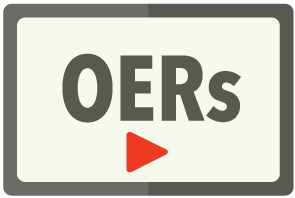## The Leading K-12 Learning Object Repository

Contains web links access to arithmetic, pre-algebra, algebra, geometry, trigonometry and calculus video mini-lessons. Topics include basic operations, integers, exponents, ratio and proportion, area, volumne, linear equations, quadratic equations, rational equations, radical equations, trigonometric functions, probability, statistics, limits, derivatives and integrals.• Requires SAFARI Montage v5.7+
• Content will be distributed online; in some cases, access to new content may require the purchase of a new content drive

## Web Proxy

Back to OERsIncluded with the purchase of any SAFARI Montage Core Content Packages.Contains web links access to arithmetic, pre-algebra, algebra, geometry, trigonometry and calculus video mini-lessons. Topics include basic operations, integers, exponents, ratio and proportion, area, volumne, linear equations, quadratic equations, rational equations, radical equations, trigonometric functions, probability, statistics, limits, derivatives and integrals.• Requires SAFARI Montage v5.7
• Content will be distributed online; in some cases, access to new content may require the purchase of a new content drive